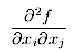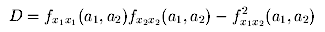#The Hessian

Prequisites: Matrices, Critical Points

Supposing we have a multi-variable function and that we have figured out its critical points; it would be nice to have a simple test to tell whether the critical points are minima, maxima, or saddle points. We find that, at least for two-variable function, that is functions of the form z=f(x,y), a fairly simple test does exist. To introduce this test, we first must define a structure called the Hessian Matrix.

Developed by Ludwig Hesse,a German mathematician, the Hessian Matrix defined for a n-variable function y= f(x1, x,2, ... xn), is the n by n matrix H whose (i,j)-th entry is the function of the second-order partial derivative

##,

a function which can be written in a more compact notation as fxixj. A technical point to notice is that the Hessian matrix is not symmetrical unless the partial drivatives fxixj are continuous. For two-variable functions, our Hessian matrix will be a 2 by 2 matrix.

Now, with all our tools in hand, let's state the test of a critical point of two variable function y= f(x1,x,2).

### The Second Derivative Test:

If f(x1,x,2) has continuous (Why is this important?) second partial derivatives in a neighborhood of a critical point (a1,a2) definef a number D by

##.

(Note that this is the determinant of f's Hessian Matrix.)

Then (a1,a2) is
1. a maximum point if D>0 and fx1x1<0
2. a minimum point if D>0 and fx1x1>0
3. a saddle point if D<0.
Further, if D=0, then no conclusion can be drawn, and any of the behaviors described above can occur.

From the above expression for D, note that if D>0, fx1x1 and fx2x2 must have the same sign.
For an explanation and justification of the above criteria and expression for D, see Explanation of the Hessian (a work in progress).

### Example:

Let's try this test on a function we've seen before, f(x,y)=x5+y4-5x-32y, which has critical points (1,2) and (-1,2). We compute
• fxx(x,y)=20x3
• fyy(x,y)=12y2
• fxy(x,y)=0
So D(x,y)=240x3y2. (Of course we quickly note to ourselves that f has continuous second partial derivatives.) Clearly for the critical point (1,2) D>0 and fxx>0 indicating (1,2) is a minimum point. On the other hand, for the critical point (-1,2) D<0 indicating (-1,2) is a saddle point. This matches our previous conclusion.

### N-Variable Functions with N>2

A natural question to ask is whether this second derivative test for two variable functions is easily generalized to higher variable functions. And the answer is "No...well, not easily anyway." Actually the two variable case is a specific case of a more general theorem, but that theorem requires a knowledge of linear algebra to understand. To see it written out, check out pp. 311 of T. M. Apostol, Calculus (John Wiley & Sons, Inc., 1969).

### Exercises:

Vector Calculus Index | World Web Math Main Page

thing@athena.mit.edu